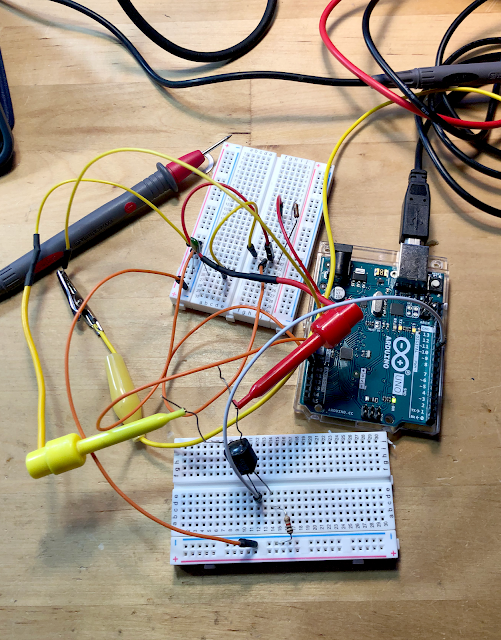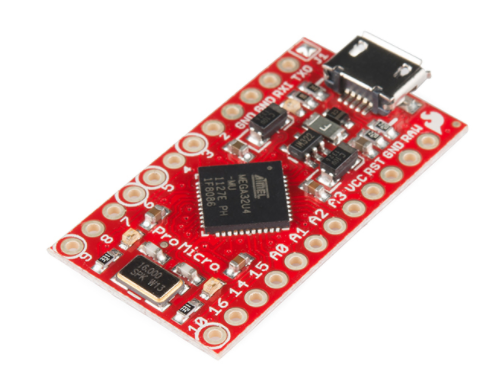## Thursday, December 26, 2019

### Vactrols Controlled by Arduinos Part II--Linear? Nope.

Hello again, from last time, can we unlock the mysterious non linearities of Vactrol/optos with the help of an Arduino?

After a few days at the bench, the answer is a qualified "probably not".

What you can do:
• You can use PWM to get different resistance values out an opto coupler aka "Vactrol" aka "vac". See the last post about this here. From that post: if you want to control a VAC with an MPU, PWM is a good way to do this.
• You can sweep through PWM values, at least lower resistance values, and record the steps into an array. The Vac will mostly behave.
• You can then do math and look ups on the array to change the VAC's behavior.
But the main problem--the deal breaker?--is the same thing that always made VACs hard to use, and something we already knew: the damn things lack linearity big time, especially when fed with low current, yielding high resistance values.

OK, After a grueling (?) + frustrating (!) 4 or so days messing with this, I mean for hours each day, my conclusion: there is way too much variation in the high-resistance range for the vacs I tested for them to ever behave in an useful, linear manner. This makes Vacs poor candidates for linear applications requiring resistance values above about 100K-200K.

Sorry.

If you want to mess with this--feel free to prove me wrong, please! read on:Uno PWM-VAC madness.....

OK, first thing to do is to make your Arduino into an Ohmmeter, preferably with a good degree of accuracy. There are a lot of posts on the web about how to do this, an easy to follow vid is here, while the most in depth pdf I could find on the subject is here.

But let's make it harder OK? I tried for two frustrating days--and I mean all day, for two damn days, to get this ohmmeter idea working on an Expressif ESP32. ESP32's can easily be set up for 15 bit PWM with a lot of easily adjustable parameters, and 12 bit A to D conversion. This makes it a perfect candidate for this project (it's also much faster than any Arduino I've tried to date).

But I couldn't get the ohmmeter part of the project working on an ESP32 with any degree of reliability. Eventually I gave up. CRAP.  I couldn't get anything better than about 80% accuracy for ohm readings, best case, and most often way, way worse. Even for 1K.  Even for 10K, even for 100K. Forget about reading vacs--that's trying to read normal resistors, using my tough-n-ready Fluke 117 to A-B what the ESP32 was coming up with. Nope, don't like it. Not one bit.

With an Arduino Uno, I could easily get 1-2% accuracy to about 100K or better just slapping in parts.Greatest thing since sliced bread--so how come simple Ohmmeter sketches didn't work?

So I used an UNO with PWM set to 256 steps and the 10 bit A-D pot.

Basic hookup is this:I used an UNO on the bench, not a Nano, and V is 5V not 5.5V.  Whatever.....

Here is the code:

//initialize pins
const int voltRead = A0;  // Analog input pin that senses Vout--used for ohm conversion

//initialize ohmmeter
int voltValue = 0;
float Vin = 5;             // Input voltage
float Vout = 0;
float buff = 0;
float buff2 = 0;
float Rref = 2000;     // Reference resistor's value in ohms
float R = 0;
float R1 = 0;
float R2 = 0;
float R3 = 0;

//pwm stuff
int x = 0;
const int pwmPin = 10;

void setup(){

pinMode(voltRead, INPUT);
pinMode(pwmPin,OUTPUT);

Serial.begin(9600);  //breakfast serial

}

void loop()
{

for (x=0;x<256;x++)
{

analogWrite(pwmPin,x);

voltValue = analogRead(voltRead);

buff = (Vin * voltValue);
Vout = (buff)/1024.0;

R1 = (Rref * Vout)/(Vin - Vout);

voltValue = analogRead(voltRead);
Serial.print("analog read is : ");
Serial.println(voltValue);
buff = (Vin * voltValue);
Vout = (buff)/1024.0;
Serial.print("x is: ");
Serial.println(x);
Serial.print("Vout is: ");
Serial.println(Vout);

R2 = (Rref * Vout)/(Vin - Vout);

voltValue = analogRead(voltRead);  // Read VAC voltage divider
Serial.print("analog read is : ");
Serial.println(voltValue);
buff = (Vin * voltValue);
Vout = (buff)/1024.0;

R3 = (Rref * Vout)/(Vin - Vout);

R=(R1 + R2 + R3)/3;

delay(1000);

Serial.print("Resistance read: ");
Serial.println(R);
}
}
//=================

(Yes I know the analog read thing can be made into a function vs repeating the code 3x. I'm too lazy. You do it.)

And yes, you get better readings if you change out the reference resistors to say 1M when you are reading high VAC resistance values. A good article about using a 4066 for this is here;  uh-huh I tried a CMOS switch for autorange; even with 300K to 1M reference the values read from the vac above 150K-300K bounce around like a coked up hamster on steroids--not usable nor stable. Reading a 1M resistor in this setup--yes. Accurate. Arduino Ohmmeter works fine even with the ref resistor pretty far off!

With a Vac--nope.How about using a DAC vs. PWM?  Tried that too! using a 4725DAC.  Easy to kludge in--info here. But--Same problem. Crappy response at high resistance readings.

OK Depending on the vac, this means we have a usable response curve from about 200K (maybe, maybe less) to about 2K, but most of the useful action is between about 10K and 2K. Is that good for your project? Probably not.

Going forward: Next thing I'd do if I were to continue this work is to put the ohmmeter values into an array, something like a[x] = R for each value of x. I messed with this too but won't bore you with all the code. If you've made it this far, you can figure the array part out.

Then the array could be sorted, you could use the map function to further goose the analog readings, or math in general could be done to elements of the array as discussed in this post.

But!!! I can't immediately think what's next for ArduinoVacLandia.

In the course of messing with this I started to think the best use case is to maybe not try to do a lookup table or a bunch of feedback to an analog pin. Instead use the PWM capabilities in an ESP32, driven by buffered and voltage divided CV; that all works. Then further tweak the incoming CV with a map function, simple addition/subtraction/division of values, and who knows what else, to get the VAC to do interesting things.

But my original idea of linearizing a vac over a large range, I think, is probably not going to happen. For that, a digital pot has got to be a better choice.

So is this the end of the story? until I bite the big one: no. I will probably revisit controlling VACs with MPUs down the road, but for now it's time to move on. I'll bet if a lot more time is spent, figuring out why the ESP32 didn't work, using a 4051 to switch between 8 reference resistors, doing a lot of math, finding some opto out there in optoland that isn't squirrely,  or whatever, yep, you can take this further.

Hopefully you have more fun than I did, because a lot of these last 3-4 days at the bench kinda sucked.

### ProMicro HID Keyboard Emulator

Quick one this time. The Arduino ProMicro (examples here and here ) is based on an Atmel 16u4 MCU and has HID keyboard emulation ready to go...